Next: Summary of the parameters Up: Basic Equations Previous: Optical Depth

Broadening is due both to the natural width of the transition and to the velocity spread of the absorbing atoms along the line of sight.
• In the ideal case of atoms at rest.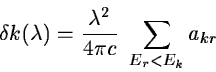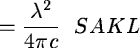• Let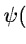be the normalized distribution of atoms with velocity between v and
v + dv, then:(8.1)

• In the program the velocity is assumed to be gaussian, thus: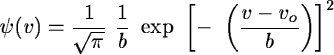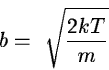vo = velocity of the cloud relative to the observer.

This full expression (1) is denoted as a Maxwell + damping wing'' or Voigtian'' profile in the program.

In the case of low column density (1)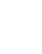can be approximated to:= NS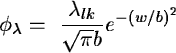(8.2)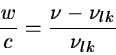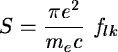This simplified expression (2) is denoted as a Maxwellian'' profile in the program.

Finally if the line of sight crosses N clouds then, the resulting optical depth is: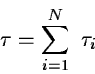In cases where the source has a (cosmological) velocity.

Let z be the redshift of the source.

An absorption is measured in the spectrum at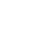=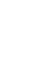corresponding to a rest wavelength.

This yields for the redshift of the cloud:The velocity of the cloud relative to the source is: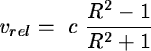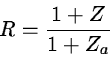In practice the program computes the absorption profile in the cloud reference frame
(vo = 0) and shifts the result into the observer's rest frame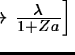Next: Summary of the parameters Up: Basic Equations Previous: Optical Depth
Petra Nass
1999-06-15